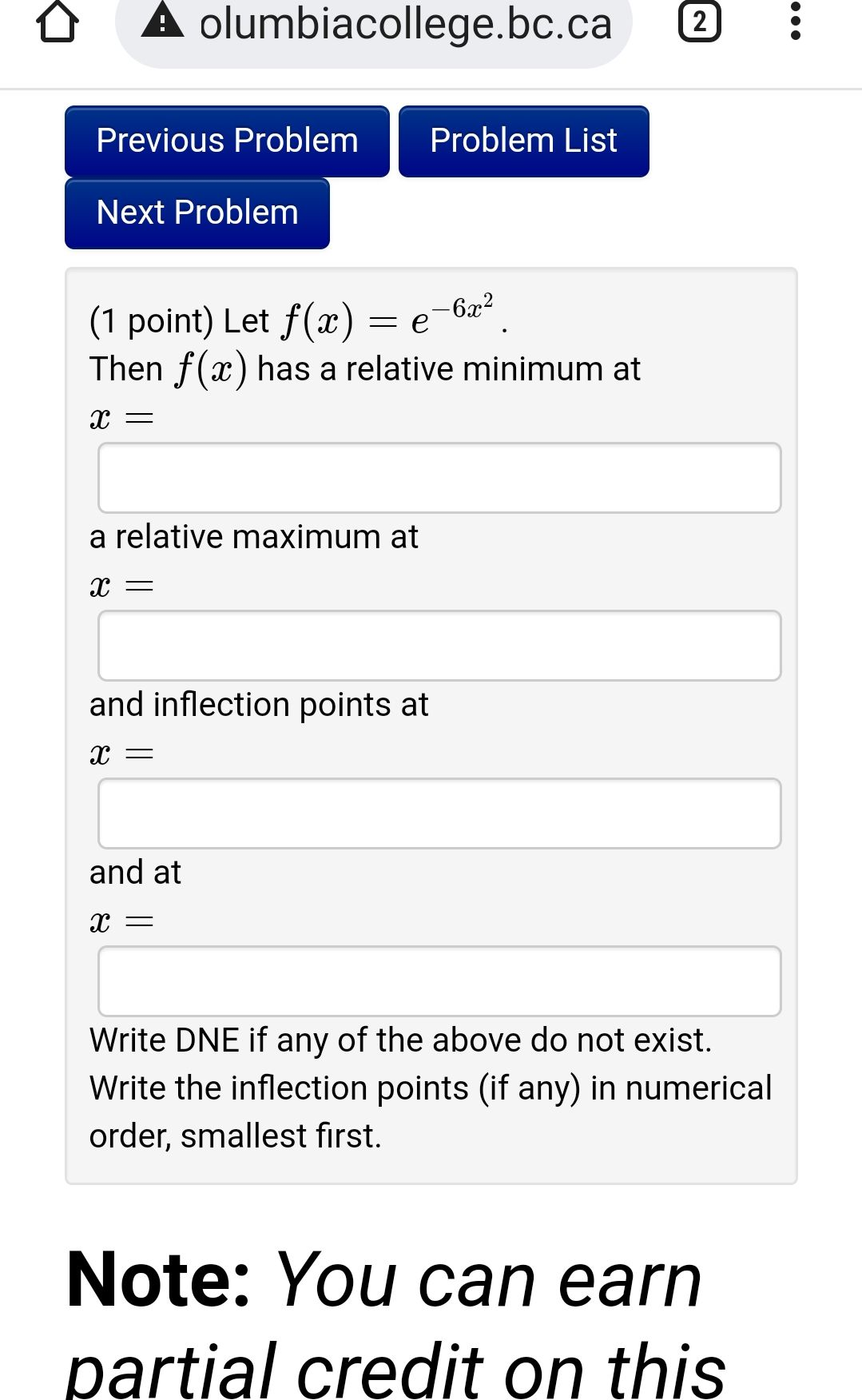### ¿Todavía tienes preguntas de matemáticas?

Pregunte a nuestros tutores expertos
Algebra
Pregunta(1 point) Let $$f ( x ) = e ^ { - 6 x ^ { 2 } }$$

Then $$f ( x )$$ has a relative minimum at

$$x =$$ a relative maximum at

$$x =$$ and inflection points at

$$x =$$

and at

$$x =$$ Write DNE if any of the above do not exist. Write the inflection points (if any) in numerical order, smallest first.

$$0;1;(0,1)$$